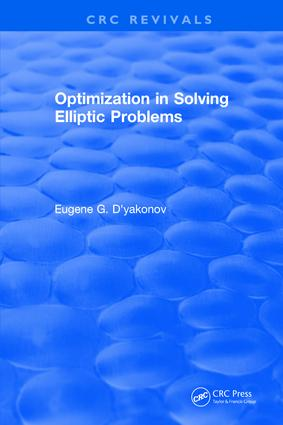# Optimization in Solving Elliptic Problems

## 1st Edition

CRC Press

590 pages

##### Purchasing Options:\$ = USD
Hardback: 9781315896113
pub: 2017-12-13
SAVE ~\$61.00
\$305.00
\$244.00
x
eBook (VitalSource) : 9781351075213
pub: 2018-05-04
from \$152.50

FREE Standard Shipping!

### Description

Optimization in Solving Elliptic Problems focuses on one of the most interesting and challenging problems of computational mathematics - the optimization of numerical algorithms for solving elliptic problems. It presents detailed discussions of how asymptotically optimal algorithms may be applied to elliptic problems to obtain numerical solutions meeting certain specified requirements. Beginning with an outline of the fundamental principles of numerical methods, this book describes how to construct special modifications of classical finite element methods such that for the arising grid systems, asymptotically optimal iterative methods can be applied.

Optimization in Solving Elliptic Problems describes the construction of computational algorithms resulting in the required accuracy of a solution and having a pre-determined computational complexity. Construction of asymptotically optimal algorithms is demonstrated for multi-dimensional elliptic boundary value problems under general conditions. In addition, algorithms are developed for eigenvalue problems and Navier-Stokes problems. The development of these algorithms is based on detailed discussions of topics that include accuracy estimates of projective and difference methods, topologically equivalent grids and triangulations, general theorems on convergence of iterative methods, mixed finite element methods for Stokes-type problems, methods of solving fourth-order problems, and methods for solving classical elasticity problems.

Furthermore, the text provides methods for managing basic iterative methods such as domain decomposition and multigrid methods. These methods, clearly developed and explained in the text, may be used to develop algorithms for solving applied elliptic problems. The mathematics necessary to understand the development of such algorithms is provided in the introductory material within the text, and common specifications of algorithms that have been developed for typical problems in mathema

### Table of Contents

1. Introduction 2. General Theory of Numerical Methods for Operator Equation s 3. Projective-Grid Methods for Second-Order Elliptic Equations and Systems 4. Estimates of Computational Work in Solving Model Grid Systems 5. Construction of Topologically Equivalent Grids 6. Asymptotic Minimization of Computational Work in Solving Second-Order Elliptic Equations and Systems 7. Estimates of Computational Work of Optimal Type for Difference Methods 8. Minimization of Computational Work for Systems of Stokes and Navier-Stokes Types 9. Asymptotically Optimal Algorithms for Fourth-Order Elliptic Problems 10. Effective Algorithms for Spectral Problems

### Subject Categories

##### BISAC Subject Codes/Headings:
MAT003000
MATHEMATICS / Applied